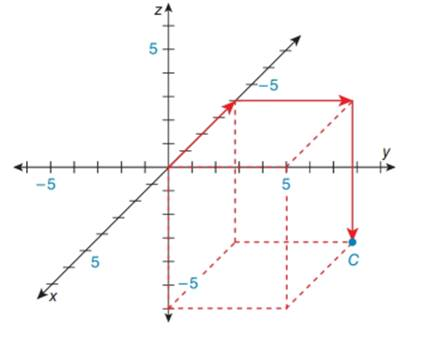Chapter 10.6, Problem 2EElementary Geometry For College St...

7th Edition
Alexander + 2 others
ISBN: 9781337614085

Solutions

Chapter
SectionElementary Geometry For College St...

7th Edition
Alexander + 2 others
ISBN: 9781337614085
Textbook Problem

In the Cartesian coordinate system below, name the ordered triple x ,   y ,   z represented by point C . Also, plot the point D 5 ,   - 4 ,   6 .To determine

To find:

The name of the ordered triple found in the given figure and plo the point C5, -4, 6.

Explanation

The given figure is shown below.

In a three dimensional Cartesian coordinate system, x-axis moves forward and backward.

Forward means positive coordinate.

Backward means negative coordinate.

y-axis moves right and left.

Right means positive coordinate.

Left means negative coordinate.

z-axis moves upward and downward.

Upward means positive coordinate.

Downward means negative coordinate.

In the given figure,

Beginning from the origin, x-axis moves 4 units backward, y-axis moves 5 units to the right and z-axis moves 6 units downwards to arrive at the given point A.

Hence, the ordered triple is C-4, 5, 6

Still sussing out bartleby?

Check out a sample textbook solution.

See a sample solution

The Solution to Your Study Problems

Bartleby provides explanations to thousands of textbook problems written by our experts, many with advanced degrees!

Get Started

Find an equation of the vertical line that passes through (0, 5).

Applied Calculus for the Managerial, Life, and Social Sciences: A Brief Approach

Show that cosh x1+12x2 for all x.

Calculus: Early Transcendentals

Proof Prove that the product of two even (or two odd) functions is even.

Calculus: Early Transcendental Functions (MindTap Course List)